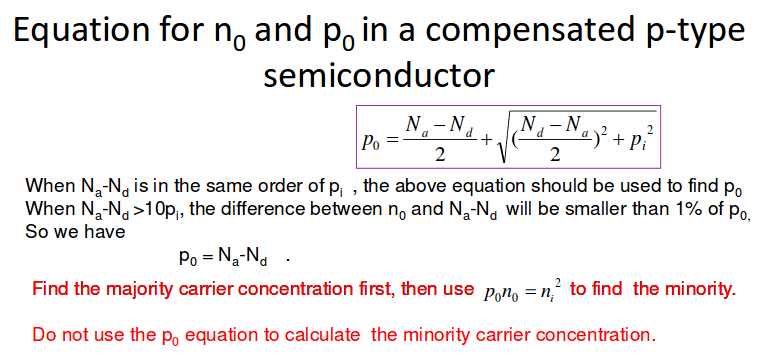# How is this equation derived?

• Turion

#### Turion

Equation in pink:I was absent so I was going through the slides and I saw that equation. There isn't a derivation shown. Do you guys have any ideas?

Last edited:
arildno said:
What course is this?

What do the symbols mean?

This is for solid state physics.

n0 represents the number of electrons in a semiconductor. p0 represents the number of holes in a semi conductor.

NA represents the number of positive (acceptor) ions. ND represents the number of negative (donor) ions.

ni=pi=n0=p0 in an instrinsic semiconductor.

If you Google "carriers doped semiconductor", 7 out of the top 7 results will lead you to a derivation. I stopped counting at that point.http://ecee.colorado.edu/~bart/book/extrinsi.htm is the first.